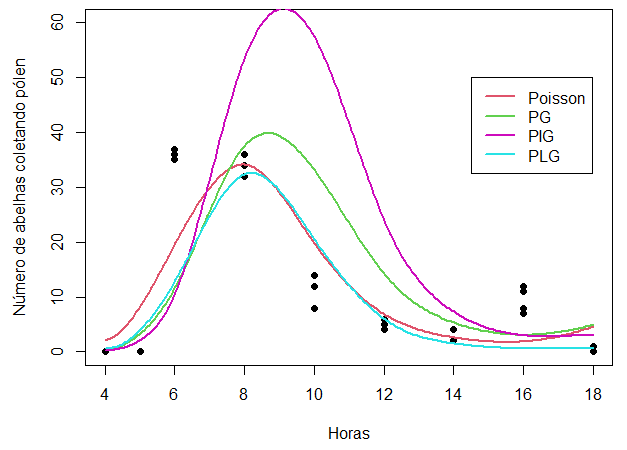# Use of Poisson, Poisson-Gamma, Poisson-Inverse Gaussian, and Generalized Poisson-Lindley distributions for count data

• Silvia Maria de Freitas Federal University of Ceará
• Caroline Gomes Duarte Departamento de Estatística e Matemática Aplicada, Universidade Federal do Ceará
Keywords: Poisson Distribution, Overdispersion, Compound Distribution, Generalized Linear Models, Hierarchical Models

### Abstract

The usual analysis for discrete data is through a Poisson, Binomial or Negative Binomial distribution, via Generalized Linear Models (GLM). However, one of the precautions to be taken when analyzing discrete data is overdispersion. This term is used when the presence of variation in the data exceeds the nominal variance stipulated by the proposed model. Thus, the use of a model based on the Poisson distribution, which assumes equidispersion, would be unfounded in the presence of overdis persion. An alternative to this problem is to use mixed distributions, through models in two stages, or hierarchical, as a way to accommodate this overdispersion. The methodology of the two-stage models as sociates a distribution to the response conditioned to its average and, later, a distribution to the average parameter, so that, unconditionally, there is a compound distribution for the response variable. In this work, the classical Poisson distribution is used for counting data, and the Gama, Inverse Gaussian and  Generalized Lindley distributions for the Poisson mean parameter, thus generating the Poisson-Gama,
35 Poisson-Inverse Gaussian and Generalized Poisson-Lindley. So, the main objective of this work is to present these hierarchical models, or models in two stages, that allow the modeling of count data with overdispersion. Futhermore, some types of residues of the structure of the MLGs were also approached, adapted for the composite distributions.

### Author Biographies

Silvia Maria de Freitas, Federal University of Ceará

Caroline Gomes Duarte, Departamento de Estatística e Matemática Aplicada, Universidade Federal do Ceará

### References

ATKINSON, A. C. Plots, transformations, and regression : an introduction to graphical methods of diagnostic regression analysis. Oxford New York: Clarendon Press Oxford University Press, 1985.

BÉLISLE, C. Convergence theorems for a class of simulated annealing algorithms on d. Journal of Applied Probability, v. 29, p. 885–895, 1992.

BICKEL, P.; DOKSUM, K. Mathematical Statistics. Oakland: Holden-day, Inc., 1977.

BRESLOW, N. Extra-poisson variation in log-linear models. Applied Statistics, v.33, 1984.

CORDEIRO, G. M.; DEMÉTRIO, C. G. B. Modelos lineares generalizados e extensões. Departamento de Estística e Informática, Recife, PE. Departamento de Ciências Exatas, USP, 2008.

COSTA, S. C. Modelos Lineares Generalizados Mistos para Dados Longitudinais. Tese (Doutorado em Agronomia) Escola Superior de Agricultura, USP, 2003.

COX, D.; SNELL, E. A general definition of residuals. Journal of the Royal Statistical Society. Series B, v.30, 07 1968.

DUNN, P. K.; SMYTH, G. K. Randomized quantile residuals. Journal of Computational and Graphical Statistics, [American Statistical Association, Taylor Francis, Ltd., Institute of Mathematical Statistics, Interface Foundation of America], v.5, n.3, p.236–244, 1996.

ELBATAL, I.; MEROVCI, F.; ELGARHY, M. A new generalized lindley distribution. Mathematical Theory and Modeling, v.3, p.30–47, 2013.

FAHRMEIR, L.; TUTZ, G. Multivariate statistical modelling based on generalized linear models. 2nd ed. Journal of the American Statistical Association, v.91, 1996.

GREENWOOD, M.; YULE, G. U. An inquiry into the nature of frequency distributions representative of multiple happenings with particular reference to the occurrence of multiple attacks of disease or of repeated accidents. Journal of the Royal Statistical Society, [Wiley, Royal Statistical Society], v. 83, n. 2, p. 255–279, 1920.

HILBE, J. M. Modeling Count Data. Cambridge University Press, 2014.

HINDE, J.; DEMETRIO, C. Overdispersion: Models and estimation. Computational Statistics Data Analysis, v. 27, p. 151–170, 1998.

HOLLA, M. On a poisson-inverse gaussian distribution. Metrika, v. 11, p. 115–121, 1967.

LAMBERT, D. Zero-inflated poisson regression, with an application to defects in manufacturing. Technometrics, v. 34, 1992.

LINDLEY, D. Fiducial distributions and bayes’ theorem. Journal of the Royal Statistical Society. Series B, v. 20, 1958.

MAHMOUDI, E.; ZAKERZADEH, H. Generalized poisson–lindley distribution. Communications in Statistics—Theory and Methods, v. 39, p. 1785–1798, 2010.

MARCIANO, F. W. P. Principais tipos de resíduos utilizados na análise de diagnóstico em MLG com aplicações para os modelos: Poisson, ZIP e ZINB, 2009.

MCCULLAGH, J. A.; NELDER, P. Generalized linear models. Boca Raton London New York: Chapman and Hall, 1989.

MENDES, A. M. F. Modelo Poisson-Lindley Generalizada para dados de contagem com superdispersão. Monografia (Graduação em Estatística) – Departamento de Estatística e Matemática Aplicada, UFC, Fortaleza, 2017.

NELDER, J.A; WEDDERBURN, R.W.M. Generalized linear models. Journal of the Royal Statistical Society. Series A (General), v.135, n.3, p.370-384, 1972.

MYERS, R.; MONTGOMERY, D.; VINING, G.; ROBINSON, T. Generalized linear models: With applications in engineering and the sciences: Second edition. 2010.

PAULA, G. A. Modelos de regressão: com apoio computacional. 2013.

POISSON, S. Probabilité des jugements en matière criminelle et en matière civile, précédées des règles générales du calcul des probabilitiés. Paris, France: Bachelier, 1837.

PUTRI, G.; NURROHMAH, s.; FITHRIANI, I. Comparing poisson-inverse gaussian model and negative binomial model on case study: horseshoe crabs data. Journal of Physics: Conference Series, 2020.

RSTUDIO TEAM. RStudio: Integrated Development Environment for R. Boston, MA, 2020. Disponível em: http://www.rstudio.com/.

SANKARAN, M. The discrete poisson-lindley distribution. Biometrics, v.26, p.145, 1970.

WONGRIN, W.; BODHISUWAN, W. The poisson-generalized lindley distribution and its applications. v. 38, p. 645–656, 2016.

ZAKERZADEH, H.; DOLATI, A. Generalized lindley distribution. Journal of Mathematical Extension, v.3, p.13–25, 2009.Published
03-03-2023
How to Cite
FreitasS. M. de, & DuarteC. G. (2023). Use of Poisson, Poisson-Gamma, Poisson-Inverse Gaussian, and Generalized Poisson-Lindley distributions for count data. Sigmae, 12(1), 172-189. Retrieved from https://publicacoes.unifal-mg.edu.br/revistas/index.php/sigmae/article/view/2077
Section
Full papers